If you're running AdBlock, please consider whitelisting this site if you'd like to support LearnOpenGL; and no worries, I won't be mad if you don't :)

Shadows are a result of the absence of light due to occlusion. When a light source's light rays do not hit an object because it gets occluded by some other object, the object is in shadow. Shadows add a great deal of realism to a lit scene and make it easier for a viewer to observe spatial relationships between objects. They give a greater sense of depth to our scene and objects. For example, take a look at the following image of a scene with and without shadows:You can see that with shadows it becomes much more obvious how the objects relate to each other. For instance, the fact that one of the cubes is floating above the others is only really noticeable when we have shadows.

Shadows are a bit tricky to implement though, specifically because in current real-time (rasterized graphics) research a perfect shadow algorithm hasn't been developed yet. There are several good shadow approximation techniques, but they all have their little quirks and annoyances which we have to take into account.

One technique used by most videogames that gives decent results and is relatively easy to implement is shadow mapping. Shadow mapping is not too difficult to understand, doesn't cost too much in performance and quite easily extends into more advanced algorithms (like Omnidirectional Shadow Maps and Cascaded Shadow Maps).

The idea behind shadow mapping is quite simple: we render the scene from the light's point of view and everything we see from the light's perspective is lit and everything we can't see must be in shadow. Imagine a floor section with a large box between itself and a light source. Since the light source will see this box and not the floor section when looking in its direction that specific floor section should be in shadow.Here all the blue lines represent the fragments that the light source can see. The occluded fragments are shown as black lines: these are rendered as being shadowed. If we were to draw a line or ray from the light source to a fragment on the right-most box we can see the ray first hits the floating container before hitting the right-most container. As a result, the floating container's fragment is lit and the right-most container's fragment is not lit and thus in shadow.

We want to get the point on the ray where it first hit an object and compare this closest point to other points on this ray. We then do a basic test to see if a test point's ray position is further down the ray than the closest point and if so, the test point must be in shadow. Iterating through possibly thousands of light rays from such a light source is an extremely inefficient approach and doesn't lend itself too well for real-time rendering. We can do something similar, but without casting light rays. Instead, we use something we're quite familiar with: the depth buffer.

You may remember from the depth testing chapter that a value in the depth buffer corresponds to the depth of a fragment clamped to [0,1] from the camera's point of view. What if we were to render the scene from the light's perspective and store the resulting depth values in a texture? This way, we can sample the closest depth values as seen from the light's perspective. After all, the depth values show the first fragment visible from the light's perspective. We store all these depth values in a texture that we call a depth map or shadow map.The left image shows a directional light source (all light rays are parallel) casting a shadow on the surface below the cube. Using the depth values stored in the depth map we find the closest point and use that to determine whether fragments are in shadow. We create the depth map by rendering the scene (from the light's perspective) using a view and projection matrix specific to that light source. This projection and view matrix together form a transformation $$T$$ that transforms any 3D position to the light's (visible) coordinate space.

A directional light doesn't have a position as it's modelled to be infinitely far away. However, for the sake of shadow mapping we need to render the scene from a light's perspective and thus render the scene from a position somewhere along the lines of the light direction.

In the right image we see the same directional light and the viewer. We render a fragment at point $$\bar{\color{red}{P}}$$ for which we have to determine whether it is in shadow. To do this, we first transform point $$\bar{\color{red}{P}}$$ to the light's coordinate space using $$T$$. Since point $$\bar{\color{red}{P}}$$ is now as seen from the light's perspective, its z coordinate corresponds to its depth which in this example is 0.9. Using point $$\bar{\color{red}{P}}$$ we can also index the depth/shadow map to obtain the closest visible depth from the light's perspective, which is at point $$\bar{\color{green}{C}}$$ with a sampled depth of 0.4. Since indexing the depth map returns a depth smaller than the depth at point $$\bar{\color{red}{P}}$$ we can conclude point $$\bar{\color{red}{P}}$$ is occluded and thus in shadow.

Shadow mapping therefore consists of two passes: first we render the depth map, and in the second pass we render the scene as normal and use the generated depth map to calculate whether fragments are in shadow. It may sound a bit complicated, but as soon as we walk through the technique step-by-step it'll likely start to make sense.

## The depth map

The first pass requires us to generate a depth map. The depth map is the depth texture as rendered from the light's perspective that we'll be using for testing for shadows. Because we need to store the rendered result of a scene into a texture we're going to need framebuffers again.

First we'll create a framebuffer object for rendering the depth map:


unsigned int depthMapFBO;
glGenFramebuffers(1, &depthMapFBO);


Next we create a 2D texture that we'll use as the framebuffer's depth buffer:



unsigned int depthMap;
glGenTextures(1, &depthMap);
glBindTexture(GL_TEXTURE_2D, depthMap);
glTexImage2D(GL_TEXTURE_2D, 0, GL_DEPTH_COMPONENT,
glTexParameteri(GL_TEXTURE_2D, GL_TEXTURE_MIN_FILTER, GL_NEAREST);
glTexParameteri(GL_TEXTURE_2D, GL_TEXTURE_MAG_FILTER, GL_NEAREST);
glTexParameteri(GL_TEXTURE_2D, GL_TEXTURE_WRAP_S, GL_REPEAT);
glTexParameteri(GL_TEXTURE_2D, GL_TEXTURE_WRAP_T, GL_REPEAT);


Generating the depth map shouldn't look too complicated. Because we only care about depth values we specify the texture's formats as GL_DEPTH_COMPONENT. We also give the texture a width and height of 1024: this is the resolution of the depth map.

With the generated depth texture we can attach it as the framebuffer's depth buffer:


glBindFramebuffer(GL_FRAMEBUFFER, depthMapFBO);
glFramebufferTexture2D(GL_FRAMEBUFFER, GL_DEPTH_ATTACHMENT, GL_TEXTURE_2D, depthMap, 0);
glDrawBuffer(GL_NONE);
glBindFramebuffer(GL_FRAMEBUFFER, 0);


We only need the depth information when rendering the scene from the light's perspective so there is no need for a color buffer. A framebuffer object however is not complete without a color buffer so we need to explicitly tell OpenGL we're not going to render any color data. We do this by setting both the read and draw buffer to GL_NONE with glDrawBuffer and glReadbuffer.

With a properly configured framebuffer that renders depth values to a texture we can start the first pass: generate the depth map. When combined with the second pass, the complete rendering stage will look a bit like this:


// 1. first render to depth map
glBindFramebuffer(GL_FRAMEBUFFER, depthMapFBO);
glClear(GL_DEPTH_BUFFER_BIT);
RenderScene();
glBindFramebuffer(GL_FRAMEBUFFER, 0);
// 2. then render scene as normal with shadow mapping (using depth map)
glViewport(0, 0, SCR_WIDTH, SCR_HEIGHT);
glClear(GL_COLOR_BUFFER_BIT | GL_DEPTH_BUFFER_BIT);
glBindTexture(GL_TEXTURE_2D, depthMap);
RenderScene();


This code left out some details, but it'll give you the general idea of shadow mapping. What is important to note here are the calls to glViewport. Because shadow maps often have a different resolution compared to what we originally render the scene in (usually the window resolution), we need to change the viewport parameters to accommodate for the size of the shadow map. If we forget to update the viewport parameters, the resulting depth map will be either incomplete or too small.

### Light space transform

An unknown in the previous snippet of code is the ConfigureShaderAndMatrices function. In the second pass this is business as usual: make sure proper projection and view matrices are set, and set the relevant model matrices per object. However, in the first pass we need to use a different projection and view matrix to render the scene from the light's point of view.

Because we're modelling a directional light source, all its light rays are parallel. For this reason, we're going to use an orthographic projection matrix for the light source where there is no perspective deform:


float near_plane = 1.0f, far_plane = 7.5f;
glm::mat4 lightProjection = glm::ortho(-10.0f, 10.0f, -10.0f, 10.0f, near_plane, far_plane);


Here is an example orthographic projection matrix as used in this chapter's demo scene. Because a projection matrix indirectly determines the range of what is visible (e.g. what is not clipped) you want to make sure the size of the projection frustum correctly contains the objects you want to be in the depth map. When objects or fragments are not in the depth map they will not produce shadows.

To create a view matrix to transform each object so they're visible from the light's point of view, we're going to use the infamous glm::lookAt function; this time with the light source's position looking at the scene's center.


glm::mat4 lightView = glm::lookAt(glm::vec3(-2.0f, 4.0f, -1.0f),
glm::vec3( 0.0f, 0.0f,  0.0f),
glm::vec3( 0.0f, 1.0f,  0.0f));


Combining these two gives us a light space transformation matrix that transforms each world-space vector into the space as visible from the light source; exactly what we need to render the depth map.


glm::mat4 lightSpaceMatrix = lightProjection * lightView;


This lightSpaceMatrix is the transformation matrix that we earlier denoted as $$T$$. With this lightSpaceMatrix, we can render the scene as usual as long as we give each shader the light-space equivalents of the projection and view matrices. However, we only care about depth values and not all the expensive fragment (lighting) calculations. To save performance we're going to use a different, but much simpler shader for rendering to the depth map.

### Render to depth map

When we render the scene from the light's perspective we'd much rather use a simple shader that only transforms the vertices to light space and not much more. For such a simple shader called simpleDepthShader we'll use the following vertex shader:


#version 330 core
layout (location = 0) in vec3 aPos;

uniform mat4 lightSpaceMatrix;
uniform mat4 model;

void main()
{
gl_Position = lightSpaceMatrix * model * vec4(aPos, 1.0);
}


This vertex shader takes a per-object model, a vertex, and transforms all vertices to light space using lightSpaceMatrix.

Since we have no color buffer and disabled the draw and read buffers, the resulting fragments do not require any processing so we can simply use an empty fragment shader:


#version 330 core

void main()
{
// gl_FragDepth = gl_FragCoord.z;
}


This empty fragment shader does no processing whatsoever, and at the end of its run the depth buffer is updated. We could explicitly set the depth by uncommenting its one line, but this is effectively what happens behind the scene anyways.

Rendering the depth/shadow map now effectively becomes:


glUniformMatrix4fv(lightSpaceMatrixLocation, 1, GL_FALSE, glm::value_ptr(lightSpaceMatrix));

glBindFramebuffer(GL_FRAMEBUFFER, depthMapFBO);
glClear(GL_DEPTH_BUFFER_BIT);
glBindFramebuffer(GL_FRAMEBUFFER, 0);


Here the RenderScene function takes a shader program, calls all relevant drawing functions and sets the corresponding model matrices where necessary.

The result is a nicely filled depth buffer holding the closest depth of each visible fragment from the light's perspective. By rendering this texture onto a 2D quad that fills the screen (similar to what we did in the post-processing section at the end of the framebuffers chapter) we get something like this: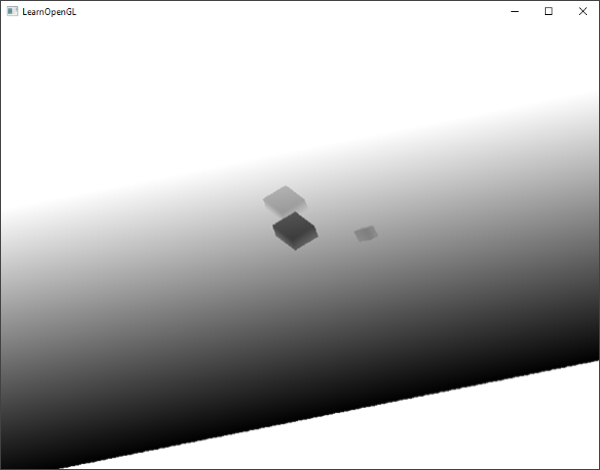For rendering the depth map onto a quad we used the following fragment shader:


#version 330 core
out vec4 FragColor;

in vec2 TexCoords;

uniform sampler2D depthMap;

void main()
{
float depthValue = texture(depthMap, TexCoords).r;
FragColor = vec4(vec3(depthValue), 1.0);
}


Note that there are some subtle changes when displaying depth using a perspective projection matrix instead of an orthographic projection matrix as depth is non-linear when using perspective projection. At the end of this chapter we'll discuss some of these subtle differences.

You can find the source code for rendering a scene to a depth map here.

With a properly generated depth map we can start rendering the actual shadows. The code to check if a fragment is in shadow is (quite obviously) executed in the fragment shader, but we do the light-space transformation in the vertex shader:


#version 330 core
layout (location = 0) in vec3 aPos;
layout (location = 1) in vec3 aNormal;
layout (location = 2) in vec2 aTexCoords;

out VS_OUT {
vec3 FragPos;
vec3 Normal;
vec2 TexCoords;
vec4 FragPosLightSpace;
} vs_out;

uniform mat4 projection;
uniform mat4 view;
uniform mat4 model;
uniform mat4 lightSpaceMatrix;

void main()
{
vs_out.FragPos = vec3(model * vec4(aPos, 1.0));
vs_out.Normal = transpose(inverse(mat3(model))) * aNormal;
vs_out.TexCoords = aTexCoords;
vs_out.FragPosLightSpace = lightSpaceMatrix * vec4(vs_out.FragPos, 1.0);
gl_Position = projection * view * vec4(vs_out.FragPos, 1.0);
}


What is new here is the extra output vector FragPosLightSpace. We take the same lightSpaceMatrix (used to transform vertices to light space in the depth map stage) and transform the world-space vertex position to light space for use in the fragment shader.

The main fragment shader we'll use to render the scene uses the Blinn-Phong lighting model. Within the fragment shader we then calculate a shadow value that is either 1.0 when the fragment is in shadow or 0.0 when not in shadow. The resulting diffuse and specular components are then multiplied by this shadow component. Because shadows are rarely completely dark (due to light scattering) we leave the ambient component out of the shadow multiplications.


#version 330 core
out vec4 FragColor;

in VS_OUT {
vec3 FragPos;
vec3 Normal;
vec2 TexCoords;
vec4 FragPosLightSpace;
} fs_in;

uniform sampler2D diffuseTexture;

uniform vec3 lightPos;
uniform vec3 viewPos;

{
[...]
}

void main()
{
vec3 color = texture(diffuseTexture, fs_in.TexCoords).rgb;
vec3 normal = normalize(fs_in.Normal);
vec3 lightColor = vec3(1.0);
// ambient
vec3 ambient = 0.15 * color;
// diffuse
vec3 lightDir = normalize(lightPos - fs_in.FragPos);
float diff = max(dot(lightDir, normal), 0.0);
vec3 diffuse = diff * lightColor;
// specular
vec3 viewDir = normalize(viewPos - fs_in.FragPos);
float spec = 0.0;
vec3 halfwayDir = normalize(lightDir + viewDir);
spec = pow(max(dot(normal, halfwayDir), 0.0), 64.0);
vec3 specular = spec * lightColor;
vec3 lighting = (ambient + (1.0 - shadow) * (diffuse + specular)) * color;

FragColor = vec4(lighting, 1.0);
}


The fragment shader is largely a copy from what we used in the advanced lighting chapter, but with an added shadow calculation. We declared a function ShadowCalculation that does most of the shadow work. At the end of the fragment shader, we multiply the diffuse and specular contributions by the inverse of the shadow component e.g. how much the fragment is not in shadow. This fragment shader takes as extra input the light-space fragment position and the depth map generated from the first render pass.

The first thing to do to check whether a fragment is in shadow, is transform the light-space fragment position in clip-space to normalized device coordinates. When we output a clip-space vertex position to gl_Position in the vertex shader, OpenGL automatically does a perspective divide e.g. transform clip-space coordinates in the range [-w,w] to [-1,1] by dividing the x, y and z component by the vector's w component. As the clip-space FragPosLightSpace is not passed to the fragment shader through gl_Position, we have to do this perspective divide ourselves:


{
// perform perspective divide
vec3 projCoords = fragPosLightSpace.xyz / fragPosLightSpace.w;
[...]
}


This returns the fragment's light-space position in the range [-1,1].

When using an orthographic projection matrix the w component of a vertex remains untouched so this step is actually quite meaningless. However, it is necessary when using perspective projection so keeping this line ensures it works with both projection matrices.

Because the depth from the depth map is in the range [0,1] and we also want to use projCoords to sample from the depth map, we transform the NDC coordinates to the range [0,1]:


projCoords = projCoords * 0.5 + 0.5;


With these projected coordinates we can sample the depth map as the resulting [0,1] coordinates from projCoords directly correspond to the transformed NDC coordinates from the first render pass. This gives us the closest depth from the light's point of view:




To get the current depth at this fragment we simply retrieve the projected vector's z coordinate which equals the depth of this fragment from the light's perspective.


float currentDepth = projCoords.z;


The actual comparison is then simply a check whether currentDepth is higher than closestDepth and if so, the fragment is in shadow:


float shadow = currentDepth > closestDepth  ? 1.0 : 0.0;


The complete ShadowCalculation function then becomes:


{
// perform perspective divide
vec3 projCoords = fragPosLightSpace.xyz / fragPosLightSpace.w;
// transform to [0,1] range
projCoords = projCoords * 0.5 + 0.5;
// get closest depth value from light's perspective (using [0,1] range fragPosLight as coords)
// get depth of current fragment from light's perspective
float currentDepth = projCoords.z;
// check whether current frag pos is in shadow
float shadow = currentDepth > closestDepth  ? 1.0 : 0.0;

}


Activating this shader, binding the proper textures, and activating the default projection and view matrices in the second render pass should give you a result similar to the image below: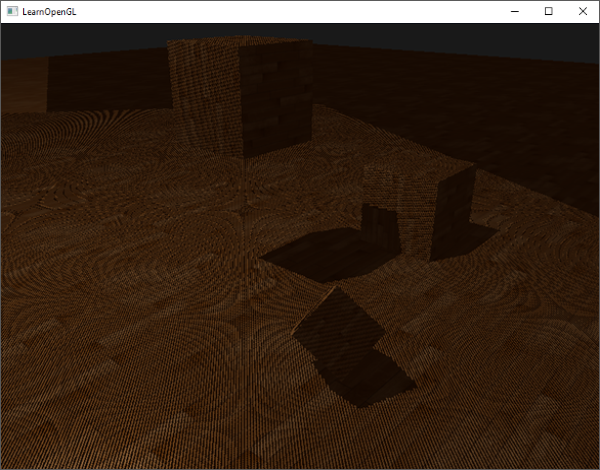If you did things right you should indeed see (albeit with quite a few artifacts) shadows on the floor and the cubes. You can find the source code of the demo application here.

We managed to get the basics of shadow mapping working, but as you can we're not there yet due to several (clearly visible) artifacts related to shadow mapping we need to fix. We'll focus on fixing these artifacts in the next sections.

It is obvious something is wrong from the previous image. A closer zoom shows us a very obvious Moiré-like pattern: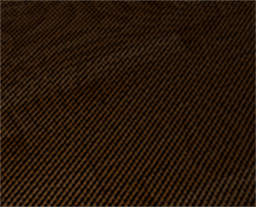We can see a large part of the floor quad rendered with obvious black lines in an alternating fashion. This shadow mapping artifact is called shadow acne and can be explained by the following image:Because the shadow map is limited by resolution, multiple fragments can sample the same value from the depth map when they're relatively far away from the light source. The image shows the floor where each yellow tilted panel represents a single texel of the depth map. As you can see, several fragments sample the same depth sample.

While this is generally okay, it becomes an issue when the light source looks at an angle towards the surface as in that case the depth map is also rendered from an angle. Several fragments then access the same tilted depth texel while some are above and some below the floor; we get a shadow discrepancy. Because of this, some fragments are considered to be in shadow and some are not, giving the striped pattern from the image.

We can solve this issue with a small little hack called a shadow bias where we simply offset the depth of the surface (or the shadow map) by a small bias amount such that the fragments are not incorrectly considered above the surface.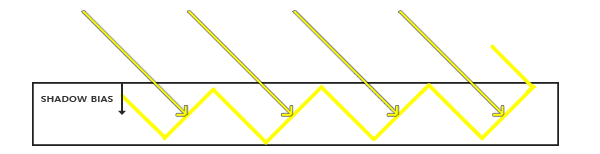With the bias applied, all the samples get a depth smaller than the surface's depth and thus the entire surface is correctly lit without any shadows. We can implement such a bias as follows:


float bias = 0.005;
float shadow = currentDepth - bias > closestDepth  ? 1.0 : 0.0;


A shadow bias of 0.005 solves the issues of our scene by a large extent, but you can imagine the bias value is highly dependent on the angle between the light source and the surface. If the surface would have a steep angle to the light source, the shadows may still display shadow acne. A more solid approach would be to change the amount of bias based on the surface angle towards the light: something we can solve with the dot product:


float bias = max(0.05 * (1.0 - dot(normal, lightDir)), 0.005);


Here we have a maximum bias of 0.05 and a minimum of 0.005 based on the surface's normal and light direction. This way, surfaces like the floor that are almost perpendicular to the light source get a small bias, while surfaces like the cube's side-faces get a much larger bias. The following image shows the same scene but now with a shadow bias:Choosing the correct bias value(s) requires some tweaking as this will be different for each scene, but most of the time it's simply a matter of slowly incrementing the bias until all acne is removed.

### Peter panning

A disadvantage of using a shadow bias is that you're applying an offset to the actual depth of objects. As a result, the bias may become large enough to see a visible offset of shadows compared to the actual object locations as you can see below (with an exaggerated bias value):This shadow artifact is called peter panning since objects seem slightly detached from their shadows. We can use a little trick to solve most of the peter panning issue by using front face culling when rendering the depth map. You may remember from the face culling chapter that OpenGL by default culls back-faces. By telling OpenGL we want to cull front faces during the shadow map stage we're switching that order around.

Because we only need depth values for the depth map it shouldn't matter for solid objects whether we take the depth of their front faces or their back faces. Using their back face depths doesn't give wrong results as it doesn't matter if we have shadows inside objects; we can't see there anyways.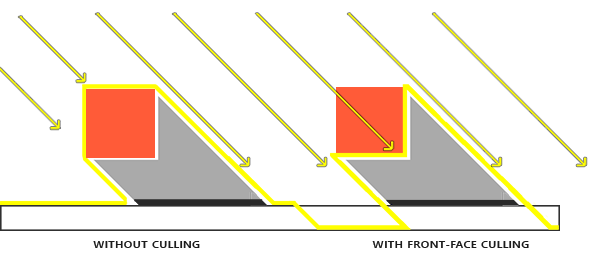To fix peter panning we cull all front faces during the shadow map generation. Note that you need to enable GL_CULL_FACE first.


glCullFace(GL_FRONT);
RenderSceneToDepthMap();
glCullFace(GL_BACK); // don't forget to reset original culling face


This effectively solves the peter panning issues, but only for solid objects that actually have an inside without openings. In our scene for example, this works perfectly fine on the cubes. However, on the floor it won't work as well as culling the front face completely removes the floor from the equation. The floor is a single plane and would thus be completely culled. If one wants to solve peter panning with this trick, care has to be taken to only cull the front faces of objects where it makes sense.

Another consideration is that objects that are close to the shadow receiver (like the distant cube) may still give incorrect results. However, with normal bias values you can generally avoid peter panning.

### Over sampling

Another visual discrepancy which you may like or dislike is that regions outside the light's visible frustum are considered to be in shadow while they're (usually) not. This happens because projected coordinates outside the light's frustum are higher than 1.0 and will thus sample the depth texture outside its default range of [0,1]. Based on the texture's wrapping method, we will get incorrect depth results not based on the real depth values from the light source.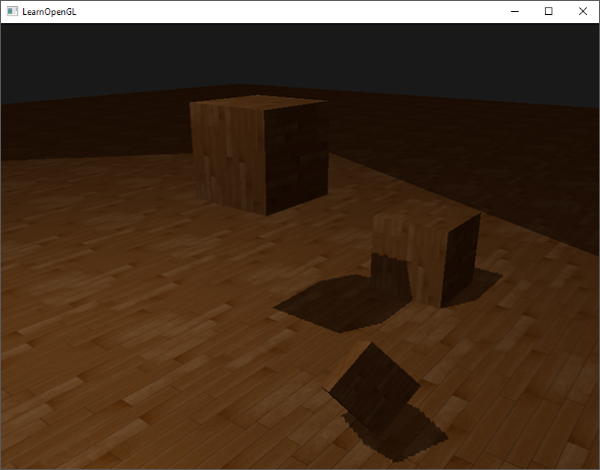You can see in the image that there is some sort of imaginary region of light, and a large part outside this area is in shadow; this area represents the size of the depth map projected onto the floor. The reason this happens is that we earlier set the depth map's wrapping options to GL_REPEAT.

What we'd rather have is that all coordinates outside the depth map's range have a depth of 1.0 which as a result means these coordinates will never be in shadow (as no object will have a depth larger than 1.0). We can do this by configuring a texture border color and set the depth map's texture wrap options to GL_CLAMP_TO_BORDER:


glTexParameteri(GL_TEXTURE_2D, GL_TEXTURE_WRAP_S, GL_CLAMP_TO_BORDER);
glTexParameteri(GL_TEXTURE_2D, GL_TEXTURE_WRAP_T, GL_CLAMP_TO_BORDER);
float borderColor[] = { 1.0f, 1.0f, 1.0f, 1.0f };
glTexParameterfv(GL_TEXTURE_2D, GL_TEXTURE_BORDER_COLOR, borderColor);


Now whenever we sample outside the depth map's [0,1] coordinate range, the texture function will always return a depth of 1.0, producing a shadow value of 0.0. The result now looks more plausible:There seems to still be one part showing a dark region. Those are the coordinates outside the far plane of the light's orthographic frustum. You can see that this dark region always occurs at the far end of the light source's frustum by looking at the shadow directions.

A light-space projected fragment coordinate is further than the light's far plane when its z coordinate is larger than 1.0. In that case the GL_CLAMP_TO_BORDER wrapping method doesn't work anymore as we compare the coordinate's z component with the depth map values; this always returns true for z larger than 1.0.

The fix for this is also relatively easy as we simply force the shadow value to 0.0 whenever the projected vector's z coordinate is larger than 1.0:


{
[...]
if(projCoords.z > 1.0)

}


Checking the far plane and clamping the depth map to a manually specified border color solves the over-sampling of the depth map. This finally gives us the result we are looking for: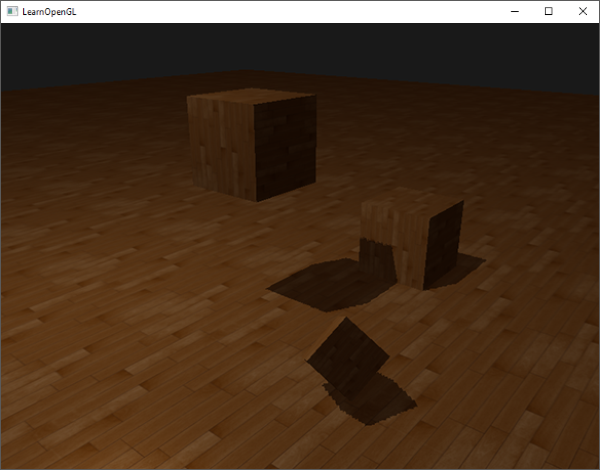The result of all this does mean that we only have shadows where the projected fragment coordinates sit inside the depth map range so anything outside the light frustum will have no visible shadows. As games usually make sure this only occurs in the distance it is a much more plausible effect than the obvious black regions we had before.

## PCF

The shadows right now are a nice addition to the scenery, but it's still not exactly what we want. If you were to zoom in on the shadows the resolution dependency of shadow mapping quickly becomes apparent.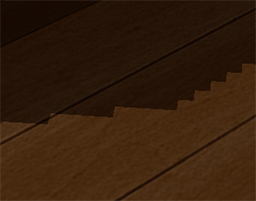Because the depth map has a fixed resolution, the depth frequently usually spans more than one fragment per texel. As a result, multiple fragments sample the same depth value from the depth map and come to the same shadow conclusions, which produces these jagged blocky edges.

You can reduce these blocky shadows by increasing the depth map resolution, or by trying to fit the light frustum as closely to the scene as possible.

Another (partial) solution to these jagged edges is called PCF, or percentage-closer filtering, which is a term that hosts many different filtering functions that produce softer shadows, making them appear less blocky or hard. The idea is to sample more than once from the depth map, each time with slightly different texture coordinates. For each individual sample we check whether it is in shadow or not. All the sub-results are then combined and averaged and we get a nice soft looking shadow.

One simple implementation of PCF is to simply sample the surrounding texels of the depth map and average the results:


vec2 texelSize = 1.0 / textureSize(shadowMap, 0);
for(int x = -1; x <= 1; ++x)
{
for(int y = -1; y <= 1; ++y)
{
float pcfDepth = texture(shadowMap, projCoords.xy + vec2(x, y) * texelSize).r;
shadow += currentDepth - bias > pcfDepth ? 1.0 : 0.0;
}
}


Here textureSize returns a vec2 of the width and height of the given sampler texture at mipmap level 0. 1 divided over this returns the size of a single texel that we use to offset the texture coordinates, making sure each new sample samples a different depth value. Here we sample 9 values around the projected coordinate's x and y value, test for shadow occlusion, and finally average the results by the total number of samples taken.

By using more samples and/or varying the texelSize variable you can increase the quality of the soft shadows. Below you can see the shadows with simple PCF applied: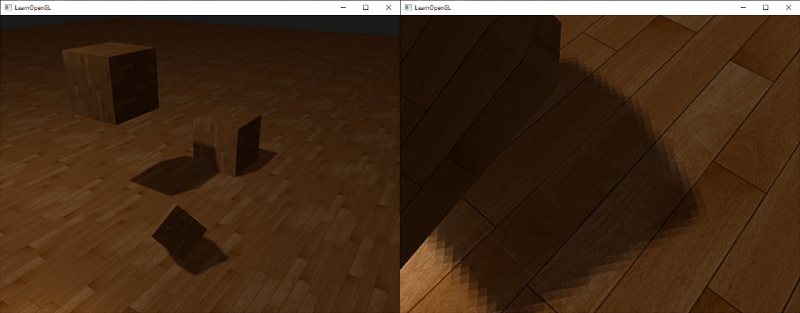From a distance the shadows look a lot better and less hard. If you zoom in you can still see the resolution artifacts of shadow mapping, but in general this gives good results for most applications.

You can find the complete source code of the example here.

There is actually much more to PCF and quite a few techniques to considerably improve the quality of soft shadows, but for the sake of this chapter's length we'll leave that for a later discussion.

## Orthographic vs perspective

There is a difference between rendering the depth map with an orthographic or a perspective projection matrix. An orthographic projection matrix does not deform the scene with perspective so all view/light rays are parallel. This makes it a great projection matrix for directional lights. A perspective projection matrix however does deform all vertices based on perspective which gives different results. The following image shows the different shadow regions of both projection methods: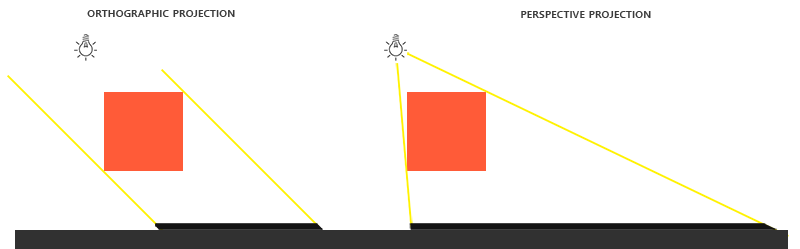Perspective projections make most sense for light sources that have actual locations, unlike directional lights. Perspective projections are most often used with spotlights and point lights, while orthographic projections are used for directional lights.

Another subtle difference with using a perspective projection matrix is that visualizing the depth buffer will often give an almost completely white result. This happens because with perspective projection the depth is transformed to non-linear depth values with most of its noticeable range close to the near plane. To be able to properly view the depth values as we did with the orthographic projection you first want to transform the non-linear depth values to linear as we discussed in the depth testing chapter:


#version 330 core
out vec4 FragColor;

in vec2 TexCoords;

uniform sampler2D depthMap;
uniform float near_plane;
uniform float far_plane;

float LinearizeDepth(float depth)
{
float z = depth * 2.0 - 1.0; // Back to NDC
return (2.0 * near_plane * far_plane) / (far_plane + near_plane - z * (far_plane - near_plane));
}

void main()
{
float depthValue = texture(depthMap, TexCoords).r;
FragColor = vec4(vec3(LinearizeDepth(depthValue) / far_plane), 1.0); // perspective
// FragColor = vec4(vec3(depthValue), 1.0); // orthographic
}


This shows depth values similar to what we've seen with orthographic projection. Note that this is only useful for debugging; the depth checks remain the same with orthographic or projection matrices as the relative depths do not change.